Courses

Test: MCQs (One or More Correct Option): Current Electricity | JEE Advanced

11 Questions MCQ Test Physics 35 Years JEE Main & Advanced Past year Papers | Test: MCQs (One or More Correct Option): Current Electricity | JEE Advanced

Description
Attempt Test: MCQs (One or More Correct Option): Current Electricity | JEE Advanced | 11 questions in 20 minutes | Mock test for JEE preparation | Free important questions MCQ to study Physics 35 Years JEE Main & Advanced Past year Papers for JEE Exam | Download free PDF with solutions
*Multiple options can be correct
QUESTION: 1

Capacitor C1 of capacitance 1 micro-farad and capacitor C2 of capacitance 2 microfarad are separately charged fully by a common battery. The two capacitors are then separately allowed to discharge through equal resistors at time t = 0.

Solution:

KEY CONCEPT : During decay of charge in R.C. circuit, I = I0e–t/RC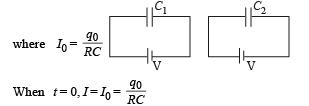Since potential difference between the plates is same initially therefore I is same in both the cases at t = 0 and is equal to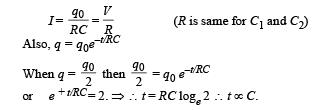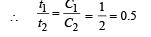or,      t1 = 0.5 t2

Therefore time taken for the first capacitor (1 µF) for discharging 50% of initial charge will be less.

*Multiple options can be correct
QUESTION: 2

Read the following statements carefully:  Y : The r esisti vity of a semicon ductor decreases with increase of temperature. Z : In a conducing solid, the rate of collisions between free electrons and ions increases with increase of temperature Select the correct statement(s) from the following;

Solution:

NOTE : The conductivity of a semiconductor increases with increase in temperature i.e. the resistivity decreases with increase in temperature.

In a conducting solid, the collisions become more frequent with increase of temperature.

*Multiple options can be correct
QUESTION: 3

In the circuit shown in Figure the current through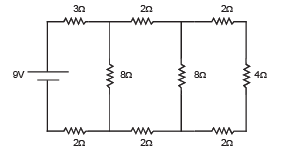Solution:

The net resistance of the circuit is 9W as shown in the following figures.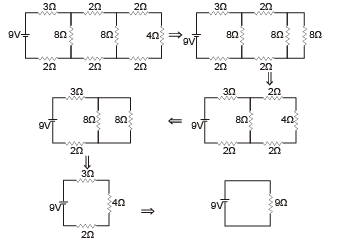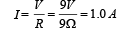The flow of current in the circuit is as follows.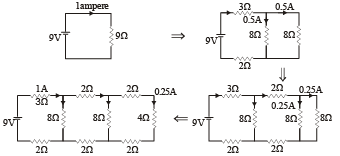NOTE : The current divides into two equal parts if passes through two equal resistances in parallel.
Thus current through 4Ω resistor is 0.25 A.

*Multiple options can be correct
QUESTION: 4

When a potential difference is applied across, the current passing through

Solution:

At 0K an insulator does not permit any current to flow through it. Option (a) is correct.
At 0K a semiconductor behaves as an insulator. Option (b) is correct.
In reverse biasing at 300 K, a very small current. flows through a p-n junction diode. Option (d) is correct.

In case of metal, the current flowing will be very-very high because a metal becomes super conductor at 0K. Option (c) is incorrect

*Multiple options can be correct
QUESTION: 5

For the circuit shown in the figure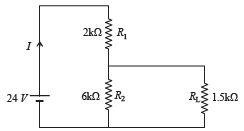Solution: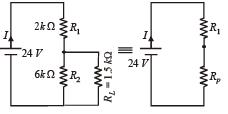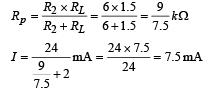⇒ option (a) is correct.
The potential difference accros RL= potential difference accros Rp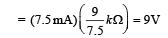option (b) is incorrect.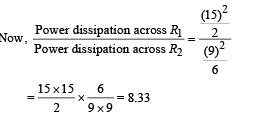option (c) is incorrect.
The magnitude of power dissipated accross R2 is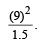Now when R1 and R2 are interchanged the equivalent resistance between R1 and RL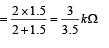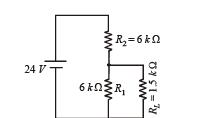∴ Potential drop across this equivalent resistance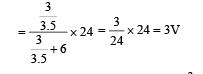∴ Potential difference accros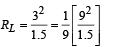∴ The magnitude of the power dissipation in RL will decrease by a factor 9 if R1 and R2 are interchanged. (d) is the correct option.

*Multiple options can be correct
QUESTION: 6

For the resistance network shown in the figure, choose the correct option(s)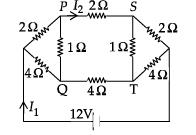Solution:

The given circuit is an extension of wheatstone bridge, therefore points P and Q are at the same potential and point S and T are also at the same potential. Therefore no current passes through PQ and ST and the circuit reduces to as shown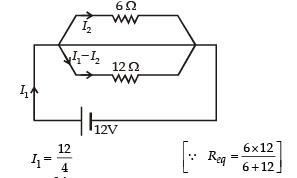= 3A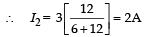As P and Q are equipotential and potential at S is less than the potential at P (potential drops across a resistance as current passes through it), therefore VS < VQ.

*Multiple options can be correct
QUESTION: 7

Heater of an electric kettle is made of a wire of length L and diameter d. It takes 4 minutes to raise the temperature of 0.5 kg water by 40 K. This heater is replaced by a new heater having two wires of the same material, each of length L and diameter 2d. The way these wires are connected is given in the options. How much time in minutes will it take to raise the temperature of the same amount of water by 40 K?

Solution: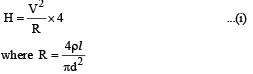When resistances are connected in series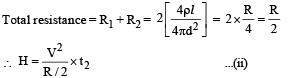From (i) and (ii) t2 = 2 min. Therefore (b) is correct.
When resistance are connected in parallel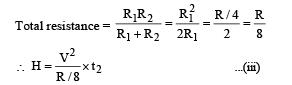From (i) and (iii) t2 = 0.5 min

∴ (d) is correct

*Multiple options can be correct
QUESTION: 8

Two ideal batteries of emf V1 and V2 and three resistances R1, R2 and R3 are connected as shown in the figure. The current in resistance R2 would be zero if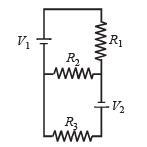Solution:

Applying KVL in MNOPQAM

V1 - iR1 + V2 - iR3=0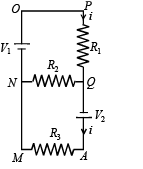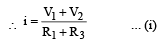Applying KVL in NOPQN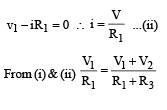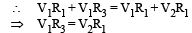If V1 = V2 then R1 = R3 = R2∴ (a) is correct option.
If V1 = V2 then R1 = R3 = 2R2∴ (b) is correct option. (R2 can have any value as there is no current flowing through d) If V1 = 2V2 then 2R3 = R1 ∴ (c) is incorrect option.
If 2V1 = V2 then R3 = 2R1 = R, ∴ (d) is correct option.

*Multiple options can be correct
QUESTION: 9

In an aluminium (Al) bar of square cross section, a square hole is drilled and is filled with iron (Fe) as shown in the figure. The electrical resistivities of Al and Fe are 2.7 × 10–8 Ω m and 1.0 × 10–7 Ω m, respectively. The electrical resistance between the two faces P and Q of the composite bar is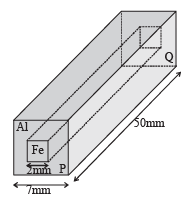Solution: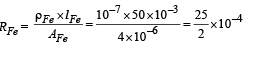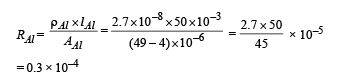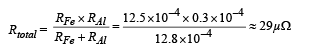*Multiple options can be correct
QUESTION: 10

An incandescent bulb has a thin filament of tungsten that is heated to high temperature by passing an electric current. The hot filament emits black–body radiation. The filament is observed to break up at random locations after a sufficiently long time of operation due to non–uniform evaporation of tungsten from the filament. If the bulb is powered at constant voltage, which of the following statement(s) is(are) true?

Solution:

With the use of filament and the evaporation involved, the filament will become thinner thereby decreasing the area of cross-section and increasing the resistance. Therefore the filament will consume less power towards the end of life.
As the evaporation is non-uniform, the area of cross-section will be different at different cross-section. Therefore temperature distribution will be non-uniform. The filament will break at the point where the temperature is maximum.
When the filament temperature is higher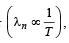it emits light of lower wavelength or higher band of frequencies.

*Multiple options can be correct
QUESTION: 11

In the circuit shown below, the key is pressed at time t = 0. Which of the following statement(s) is(are) true?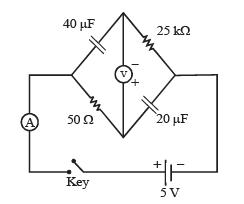Solution:

At t=0, Capacitors act as short circuit and voltmeter display –5v At t = µ, Capacitor acts as open circuit and no current flows through voltmeter ( ∴ very high resistance of voltmeter) so it display +5V. (a) is the correct option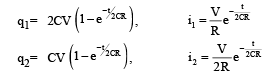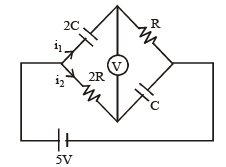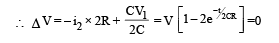(b) is the correct option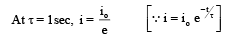∴ (c) is the correct option After  a long time no current flows since both capcitor and voltmeter do not allow current to flow.

∴ (d) is the correct option.Use Code STAYHOME200 and get INR 200 additional OFF Use Coupon Code

Track your progress, build streaks, highlight & save important lessons and more!

Similar ContentRelated tests